# deshi

Showing the single result

• ## Dals & Pulses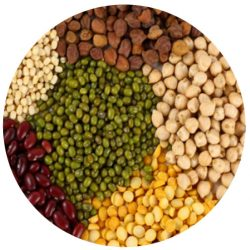26 Incl. Tax

Quick Order From our Dal and Pulses Menu, you can Blindly trust our quality. Soon you will realise why we say Accha Khao, Maze se Raho – Since 70 Years…..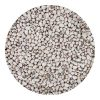Barbati Weight Choose Weight250gm - Rs. 26500gm - Rs. 481kg - Rs. 922kg - Rs. 1801.5kg - Rs. 1362.5kg - Rs. 2243kg - Rs. 268 Clear ₹26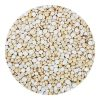Batana Daal Weight Choose Weight1kg - Rs. 922kg - Rs. 180500gm - Rs. 481.5kg - Rs. 1362.5kg - Rs. 2243kg - Rs. 268 Clear ₹48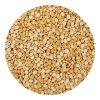Chana Daal Weight Choose Weight500gm - Rs. 421kg - Rs. 802kg - Rs. 1561.5kg - Rs. 1182.5kg - Rs. 1943kg - Rs. 232 Clear ₹42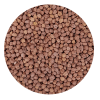Desi Chana Weight Choose Weight500gm - Rs. 361kg - Rs. 682kg - Rs. 1321.5kg - Rs. 1002.5kg - Rs. 1643kg - Rs. 196 Clear ₹36Daliya Weight Choose Weight250gm - Rs. 28500gm - Rs. 511kg - Rs. 971.5kg - Rs. 1432.5kg - Rs. 2353kg - Rs. 281 Clear ₹28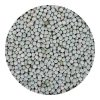Hara Batana Weight Choose Weight500gm - Rs. 371kg - Rs. 701.5kg - Rs. 1032.5kg - Rs. 1693kg - Rs. 202 Clear ₹37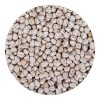Kabuli Chana Weight Choose Weight500gm - Rs. 761kg - Rs. 1482kg - Rs. 2921.5kg - Rs. 2202.5kg - Rs. 3643kg - Rs. 436 Clear ₹76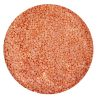Masoor Daal Weight Choose Weight200gm - Rs. 22500gm - Rs. 491kg - Rs. 942kg - Rs. 1841.5kg - Rs. 1392.5kg - Rs. 2293kg - Rs. 274 Clear ₹22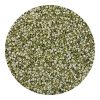Moong Daal Chilka Weight Choose Weight500gm - Rs. 661kg - Rs. 1282kg - Rs. 2521.5kg - Rs. 1902.5kg - Rs. 3143kg - Rs. 376 Clear ₹66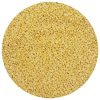Moong Mogar Weight Choose Weight500gm - Rs. 731kg - Rs. 1422kg - Rs. 2801.5kg - Rs. 2112.5kg - Rs. 3493kg - Rs. 418 Clear ₹73Moong Sabut Weight Choose Weight500gm - Rs. 691kg - Rs. 1342kg - Rs. 2641.5kg - Rs. 1992.5kg - Rs. 3293kg - Rs. 394 Clear ₹69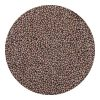Motha Weight Choose Weight500gm - Rs. 541kg - Rs. 1042kg - Rs. 2041.5kg - Rs. 1542.5kg - Rs. 2543kg - Rs. 304 Clear ₹54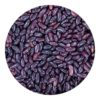Rajma Weight Choose Weight250gm - Rs. 44500gm - Rs. 841kg - Rs. 1641.5kg - Rs. 2442kg - Rs. 3243kg - Rs. 484 Clear ₹44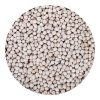Safed Batana Weight Choose Weight250gm - Rs. 23500gm - Rs. 421kg - Rs. 801.5kg - Rs. 1182.5kg - Rs. 1943kg - Rs. 232 Clear ₹23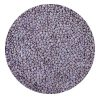Masoor Saiga Whole Weight Choose Weight250gm - Rs. 27500gm - Rs. 491kg - Rs. 941.5kg - Rs. 1392.5kg - Rs. 2293kg - Rs. 274 Clear ₹27Urad Saiga Whole Weight Choose Weight250gm - Rs. 30500gm - Rs. 561kg - Rs. 1081.5kg - Rs. 1602.5kg - Rs. 2643kg - Rs. 316 Clear ₹30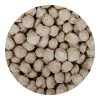Soyabean Badi Weight Choose Weight100gm - Rs. 14200gm - Rs. 24250gm - Rs. 29300gm - Rs. 34500gm - Rs. 54 Clear ₹14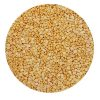Tur Daal Supreme Weight Choose Weight500gm - Rs. 731kg - Rs. 1422kg - Rs. 2801.5kg - Rs. 2112.5kg - Rs. 3493kg - Rs. 418 Clear ₹73Urad Daal Chilka Weight Choose Weight250gm - Rs. 31500gm - Rs. 571kg - Rs. 1101.5kg - Rs. 1632.5kg - Rs. 2693kg - Rs. 322 Clear ₹31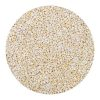Urad Daal Mogar Weight Choose Weight250gm - Rs. 33500gm - Rs. 621kg - Rs. 1202kg - Rs. 2361.5kg - Rs. 1782.5kg - Rs. 294 Clear ₹33

## Delete or Select Product from Collection by Selecting Pack type and Changing Quantity.

SKU: wb3008808 Category: